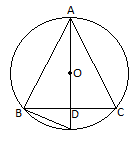Newbie

# In a circular table cover of radius 32 cm, a design is formed leaving an equilateral triangle ABC in the middle as shown in Fig. 12.24. Find the area of the design.Q6

• 0

What is the solution of ncert class 10 chapter areas related to circles , how i solve this question in a easiest and simplest way . Find the best solution of exercise 12.3 question no. 6 , sir please help me to solve this question in a easiest way.In a circular table cover of radius 32 cm, a design is formed leaving an equilateral triangle ABC in the middle as shown in Fig. 12.24. Find the area of the design

Share

1. Radius of the circle = 32 cm

Draw a median AD of the triangle passing through the centre of the circle.

⇒ BD = AB/2

Since, AD is the median of the triangleBy Pythagoras theorem,

⇒ AB= 482+(AB/2)2

⇒ AB= 2304+AB2/4

⇒ 3/4 (AB2)= 2304

⇒ AB= 3072

⇒ AB= 32√3 cm

Area of ΔADB = √3/4 ×(32√3)cm= 768√3 cm2

Area of circle = πR2 = (22/7)×32×32 = 22528/7 cm2

Area of the design = Area of circle – Area of ΔADB

= (22528/7 – 768√3) cm2

• 0